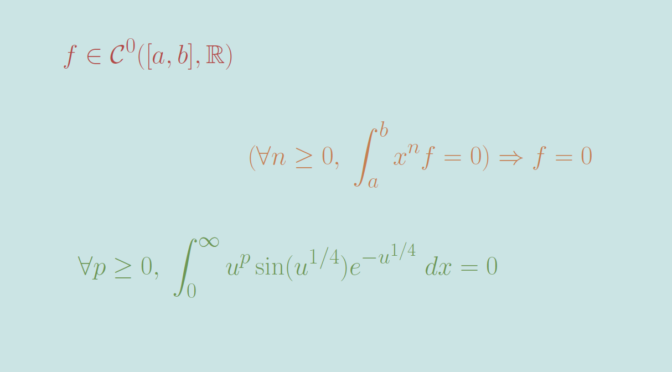A nonzero continuous map orthogonal to all polynomials

Let’s consider the vector space $$\mathcal{C}^0([a,b],\mathbb R)$$ of continuous real functions defined on a compact interval $$[a,b]$$. We can define an inner product on pairs of elements $$f,g$$ of $$\mathcal{C}^0([a,b],\mathbb R)$$ by $\langle f,g \rangle = \int_a^b f(x) g(x) \ dx.$

It is known that $$f \in \mathcal{C}^0([a,b],\mathbb R)$$ is the always vanishing function if we have $$\langle x^n,f \rangle = \int_a^b x^n f(x) \ dx = 0$$ for all integers $$n \ge 0$$. Let’s recall the proof. According to Stone-Weierstrass theorem, for all $$\epsilon >0$$ it exists a polynomial $$P$$ such that $$\Vert f – P \Vert_\infty \le \epsilon$$. Then \begin{aligned} 0 &\le \int_a^b f^2 = \int_a^b f(f-P) + \int_a^b fP\\ &= \int_a^b f(f-P) \le \Vert f \Vert_\infty \epsilon(b-a) \end{aligned} As this is true for all $$\epsilon > 0$$, we get $$\int_a^b f^2 = 0$$ and $$f = 0$$.

We now prove that the result becomes false if we change the interval $$[a,b]$$ into $$[0, \infty)$$, i.e. that one can find a continuous function $$f \in \mathcal{C}^0([0,\infty),\mathbb R)$$ such that $$\int_0^\infty x^n f(x) \ dx$$ for all integers $$n \ge 0$$. In that direction, let’s consider the complex integral $I_n = \int_0^\infty x^n e^{-(1-i)x} \ dx.$ $$I_n$$ is well defined as for $$x \in [0,\infty)$$ we have $$\vert x^n e^{-(1-i)x} \vert = x^n e^{-x}$$ and $$\int_0^\infty x^n e^{-x} \ dx$$ converges. By integration by parts, one can prove that $I_n = \frac{n!}{(1-i)^{n+1}} = \frac{(1+i)^{n+1}}{2^{n+1}} n! = \frac{e^{i \frac{\pi}{4}(n+1)}}{2^{\frac{n+1}{2}}}n!.$ Consequently, $$I_{4p+3} \in \mathbb R$$ for all $$p \ge 0$$ which means $\int_0^\infty x^{4p+3} \sin(x) e^{-x} \ dx =0$ and finally $\int_0^\infty u^p \sin(u^{1/4}) e^{-u^{1/4}} \ dx =0$ for all integers $$p \ge 0$$ using integration by substitution with $$x = u^{1/4}$$. The function $$u \mapsto \sin(u^{1/4}) e^{-u^{1/4}}$$ is one we were looking for.